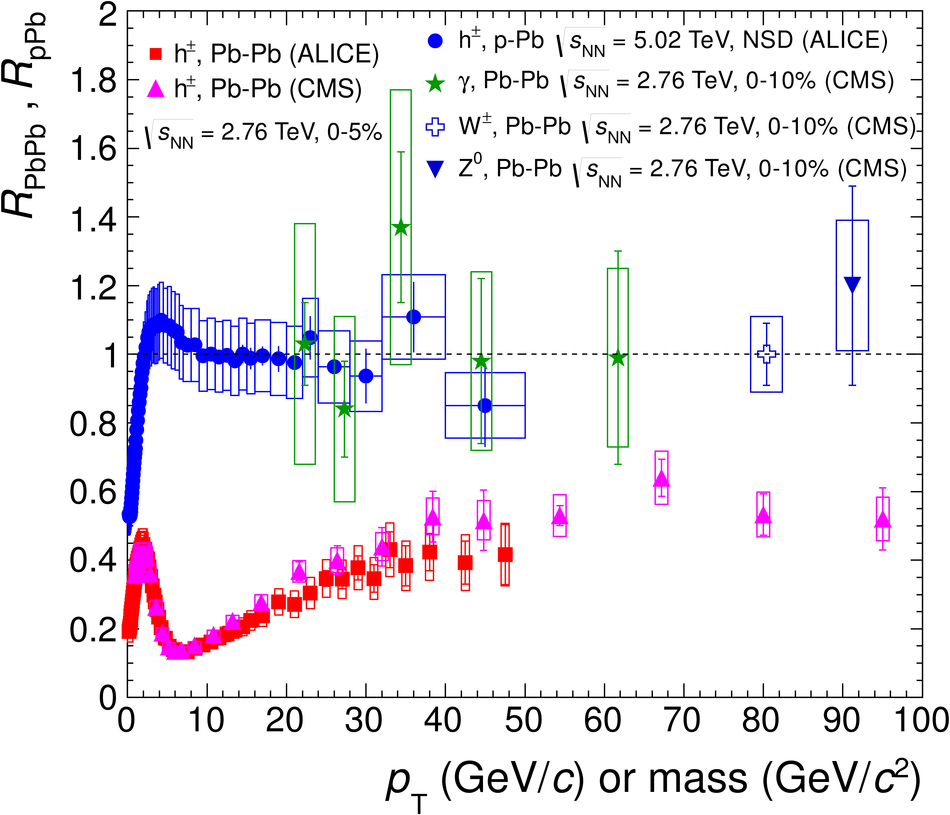# Figure 4

 Transverse momentum dependence of the nuclear modification factor $R_{\mathrm{pPb}}$ of charged particles (h$^\pm$) measured in minimum-bias (NSD) p-Pb collisions at $\sqrt{s_{\mathrm{NN}}} = 5.02$ TeV in comparison to data on the nuclear modification factor $R_{\mathrm{PbPb}}$ in central Pb-Pb collisions at $\sqrt{s_{\mathrm{NN}}} = 2.76$ TeV. The Pb-Pb data are for charged particle, direct photon, Z$^0$ and W$^{\pm}$ production. All data are for midrapidity.